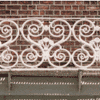#### You may also like### Frieze Patterns in Cast Iron

A gallery of beautiful photos of cast ironwork friezes in Australia with a mathematical discussion of the classification of frieze patterns.### The Frieze Tree

Patterns that repeat in a line are strangely interesting. How many types are there and how do you tell one type from another?### Friezes

Some local pupils lost a geometric opportunity recently as they surveyed the cars in the car park. Did you know that car tyres, and the wheels that they on, are a rich source of geometry?

# Reflecting Lines

##### Age 11 to 14Challenge Level

Jiwon Jung noticed:

The lines that are reflections have the same absolute value of their x coefficient and the constant.

Well noticed. Ellie from Chiswick matched up the reflections, and explained why they work:

A straight line is $y=ax+b$, where $a$ and $b$ are constants, and can be positive or negative.
If you reflect in the $x$ axis, then all the $y$ values become $-y$, so the line becomes $-y=ax+b$, which we write $y=-ax-b$.
If we reflect in the $y$ axis, then the $x$ values become $-x$, and the line becomes $y=a(-x)+b$, which is $y=-ax+b$.

Now we can match the lines.Then I looked at doing both reflections.
First, I tried reflect in $x$, then $y$, using the rules above.
$y=ax+b$ reflected in $x$ is $y=-ax-b$, then reflected in $y$ becomes $y=ax-b$
Now try the other way around.
$y=ax+b$ reflected in $y$ is $y=-ax+b$, then reflected in $x$ becomes $y=ax-b$

So it doesn't matter which way round you do the reflections. The twice reflected graph has the same gradient as the original, but translated down so it crosses the $y$ axis at the opposite place.